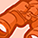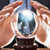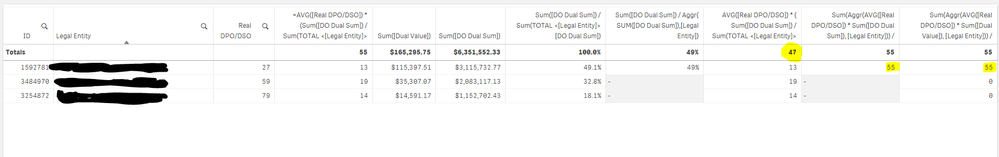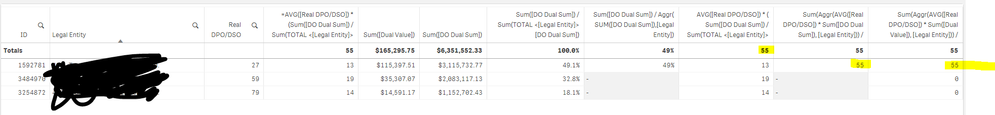New to Qlik Sense

If you’re new to Qlik Sense, start with this Discussion Board and get up-to-speed quickly.

Announcements
QlikWorld 2022, LIVE in Denver CO., May 16-19, 2022. REGISTER NOW TO RECEIVE EARLY BIRD PRICING
cancel
Showing results for
Did you mean:Contributor III

A subtotal / aggr related question | Weighted Average days per dimension

Hi,

I've the following formula that works great:

AVG([Days]) * (Sum([Value]) / Sum(TOTAL <[Dimension]> [DO Dual Sum]))

Now if I choose to use SUM as the total, instead of AUTO I get the value I want. I want to display that SUM aggregated per Dimension

So tried things like:

SUM(aggr(AVG([DSO]) * (Sum([DO Dual Sum]) / Sum(TOTAL <[Dimension]> [DO Dual Sum])),Dimension))

This however, gives me a different value.

Any idea?

Labels (1)
• subtotal

3 RepliesMVP

May be any one of the follows? Please use Auto option

Sum(Aggr(AVG([Days]) * (Sum([Value]), Dim1)) / Sum(TOTAL <[Dimension]> [DO Dual Sum])

Or

Sum(Aggr(AVG([Days]) * (Sum([Value]), Dim1)) / Sum(Aggr(Sum(TOTAL <[Dimension]> [DO Dual Sum]), Dim1))

Before develop something, think If placed (The Right information | To the right people | At the Right time | In the Right place | With the Right context)Contributor III

Unfortunately, these two options you provided give the same result as if you would have used Auto in the originally posted calculation (55) AVG( [Real DPO/DSO]) * ( Sum([DO Dual Sum]) / Sum(TOTAL <[Legal Entity]> [DO Dual Sum]) ), but we want here is to get 47.

Sum in Totals = 47Auto in Totals = 55MVP

Are you using a calculated dimension where you have restricted the dimension to be some set of the all the values within dimension? If you did, then you might need to use set analysis in your expression to get the right total.Tags
Community Browser